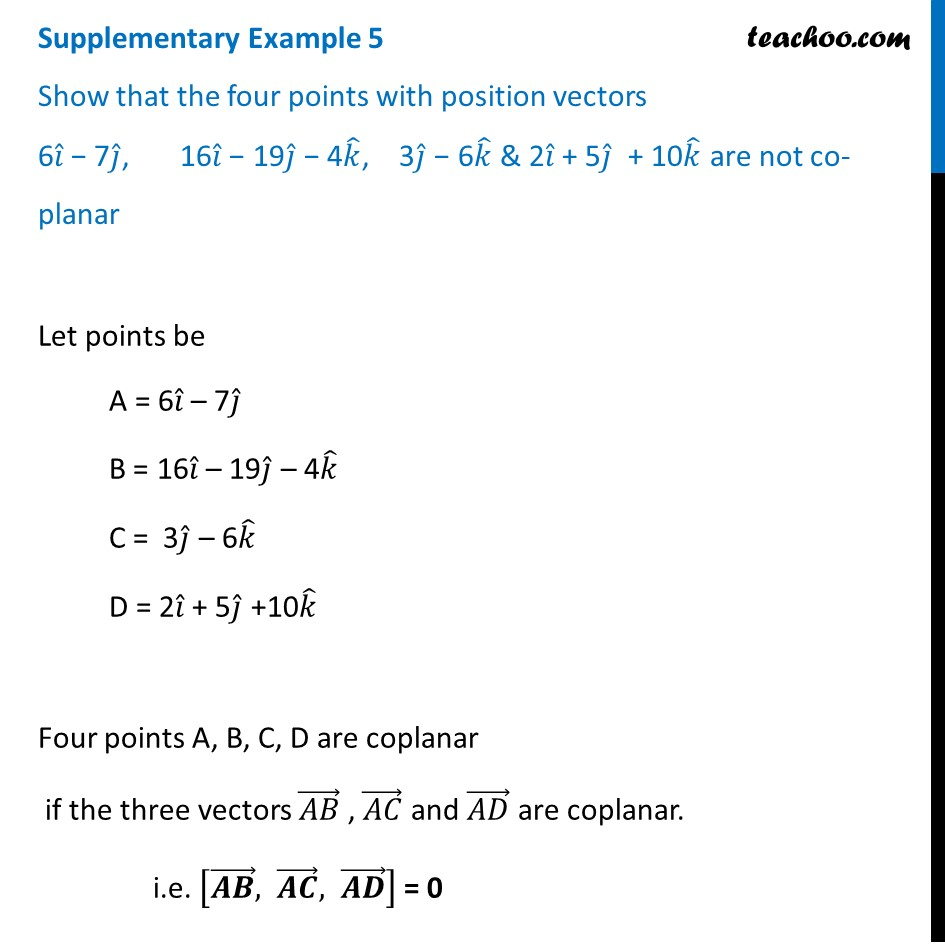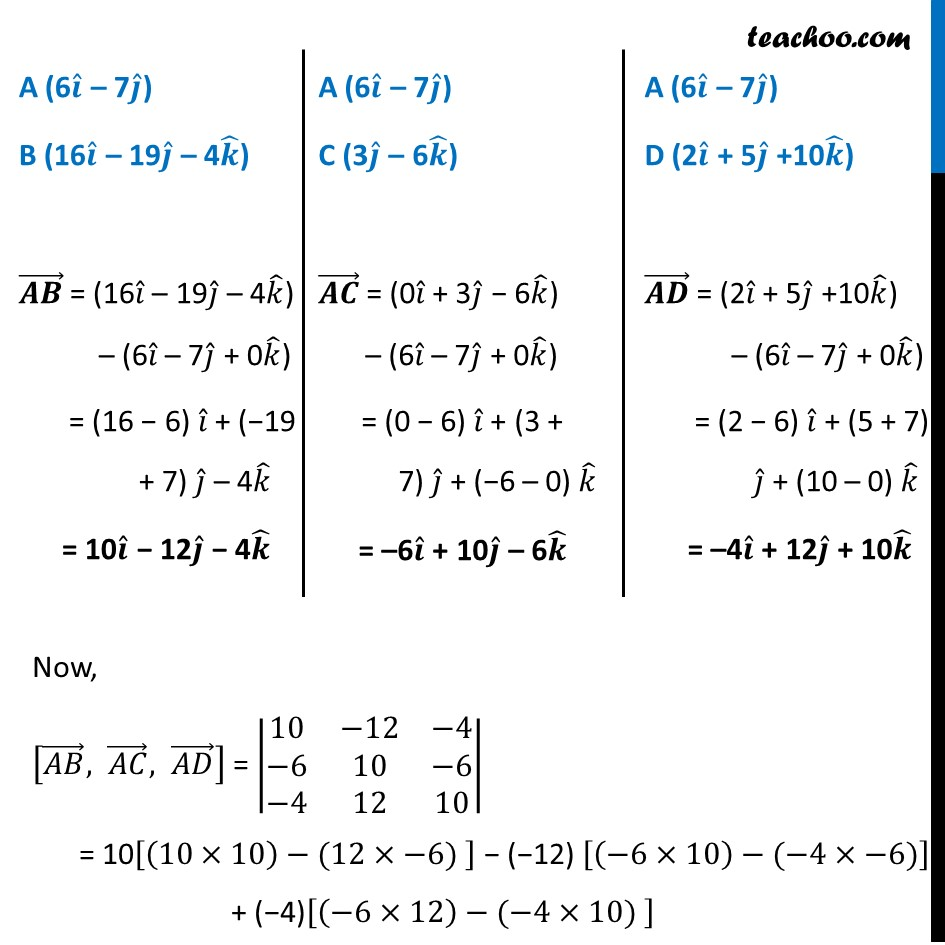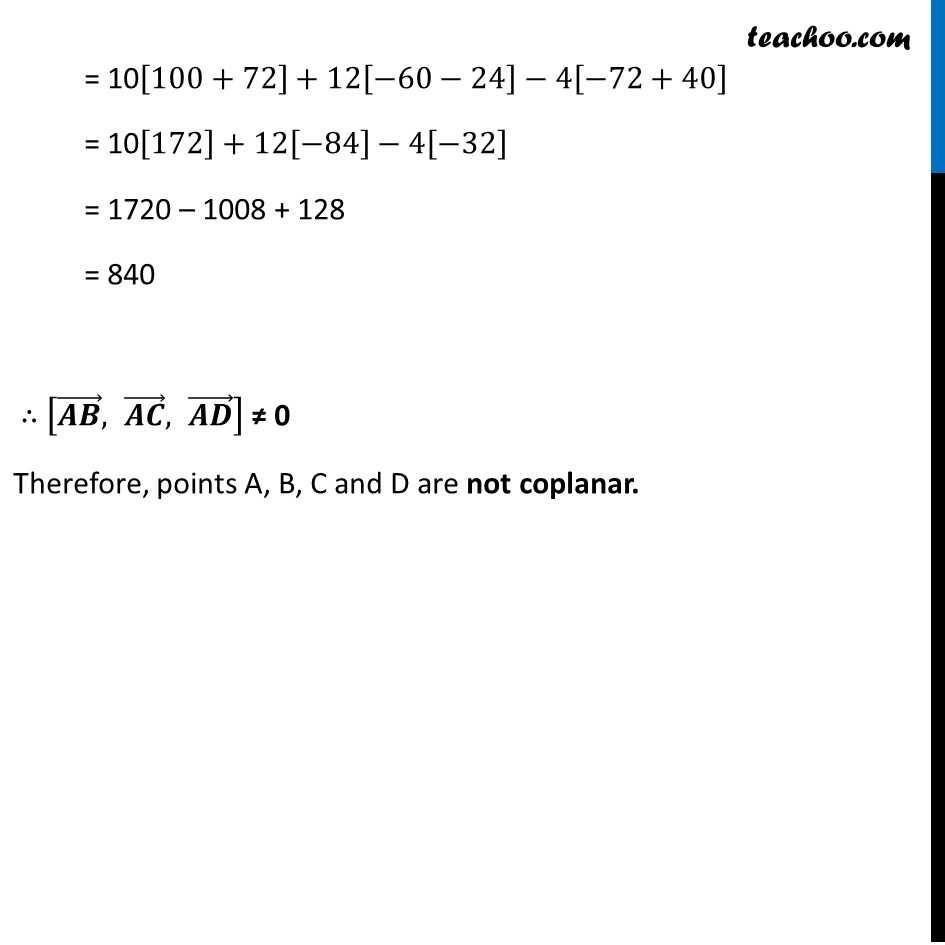Supplementary examples and questions from CBSE

Chapter 10 Class 12 Vector Algebra
Serial order wiseLearn in your speed, with individual attention - Teachoo Maths 1-on-1 Class

### Transcript

Supplementary Example 5 Show that the four points with position vectors 6𝑖 ̂ − 7𝑗 ̂, 16𝑖 ̂ − 19𝑗 ̂ − 4𝑘 ̂, 3𝑗 ̂ − 6𝑘 ̂ & 2𝑖 ̂ + 5𝑗 ̂ + 10𝑘 ̂ are not co-planar Let points be A = 6𝑖 ̂ – 7𝑗 ̂ B = 16𝑖 ̂ – 19𝑗 ̂ – 4𝑘 ̂ C = 3𝑗 ̂ – 6𝑘 ̂ D = 2𝑖 ̂ + 5𝑗 ̂ +10𝑘 ̂ Four points A, B, C, D are coplanar if the three vectors (𝐴𝐵) ⃗ , (𝐴𝐶) ⃗ and (𝐴𝐷) ⃗ are coplanar. i.e. [(𝑨𝑩) ⃗, (𝑨𝑪) ⃗, (𝑨𝑫) ⃗ ] = 0 A (6𝒊 ̂ – 7𝒋 ̂) B (16𝒊 ̂ – 19𝒋 ̂ – 4𝒌 ̂) (𝑨𝑩) ⃗ = (16𝑖 ̂ – 19𝑗 ̂ – 4𝑘 ̂) – (6𝑖 ̂ – 7𝑗 ̂ + 0𝑘 ̂) = (16 − 6) 𝑖 ̂ + (−19 + 7) 𝑗 ̂ – 4𝑘 ̂ = 10𝒊 ̂ − 12𝒋 ̂ − 4𝒌 ̂ A (6𝒊 ̂ – 7𝒋 ̂) C (3𝒋 ̂ – 6𝒌 ̂) (𝑨𝑪) ⃗ = (0𝑖 ̂ + 3𝑗 ̂ − 6𝑘 ̂) – (6𝑖 ̂ – 7𝑗 ̂ + 0𝑘 ̂) = (0 − 6) 𝑖 ̂ + (3 + 7) 𝑗 ̂ + (−6 – 0) 𝑘 ̂ = –6𝒊 ̂ + 10𝒋 ̂ – 6𝒌 ̂ A (6𝒊 ̂ – 7𝒋 ̂) D (2𝒊 ̂ + 5𝒋 ̂ +10𝒌 ̂) (𝑨𝑫) ⃗ = (2𝑖 ̂ + 5𝑗 ̂ +10𝑘 ̂) – (6𝑖 ̂ – 7𝑗 ̂ + 0𝑘 ̂) = (2 − 6) 𝑖 ̂ + (5 + 7) 𝑗 ̂ + (10 – 0) 𝑘 ̂ = –4𝒊 ̂ + 12𝒋 ̂ + 10𝒌 ̂ Now, [(𝐴𝐵) ⃗, (𝐴𝐶) ⃗, (𝐴𝐷) ⃗ ] = |■8(10&−12&−4@−6&10&−6@−4&12&10)| = 10[(10×10)−(12×−6) ] − (−12) [(−6×10)−(−4×−6)] + (−4)[(−6×12)−(−4×10) ] = 10[100+72]+12[−60−24]−4[−72+40] = 10+12[−84]−4[−32] = 1720 – 1008 + 128 = 840 ∴ [(𝑨𝑩) ⃗, (𝑨𝑪) ⃗, (𝑨𝑫) ⃗ ] ≠ 0 Therefore, points A, B, C and D are not coplanar.